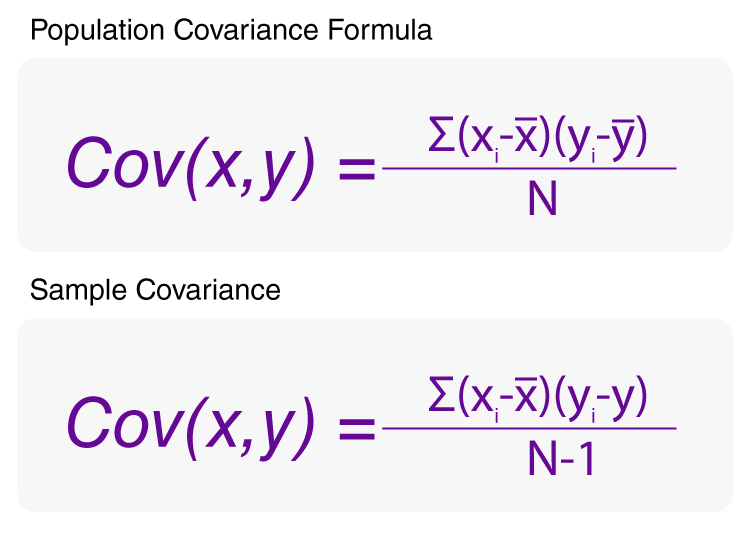# Covariance Formula

Covariance formula is a statistical formula which is used to assess the relationship between two variables. In simple words, covariance is one of the statistical measurement to know the relationship of the variance between the two variables.

The covariance indicates how two variables are related and also helps to know whether the two variables vary together or change together. The covariance is denoted as Cov(X,Y) and the formulas for covariance are given below.Formula to Find Sample and Population Covariance

## Formulas for Covariance (Population and Sample)

 Population Covariance Formula Cov(X,Y)= $$\begin{array}{l} \frac{\sum (x_{i}-\overline{x})(y_{i}-\overline{y})}{N}\end{array}$$ Sample Covariance Formula Cov(X,Y)= $$\begin{array}{l} \frac{\sum (x_{i}-\overline{x})(y_{i}-\overline{y})}{N-1}\end{array}$$

### Notations in Covariance Formulas

• xi = data value of x
• yi = data value of y
• x̄ = mean of x
• ȳ = mean of y
• N = number of data values.

### Relation Between Correlation Coefficient and Covariance Formulas

$$\begin{array}{l}Correlation = \frac{Cov(x,y)}{\sigma_x*\sigma_y}\end{array}$$

Here, Cov (x,y) is the covariance between x and y while σx and σy are the standard deviations of x and y. Using the above formula, the correlation coefficient formula can be derived using the covariance and vice versa.

### Example Question Using Covariance Formula

Question: The table below describes the rate of economic growth (xi) and the rate of return on the S&P 500 (yi). Using the covariance formula, determine whether economic growth and S&P 500 returns have a positive or inverse relationship. Before you compute the covariance, calculate the mean of x and y.

Economic Growth % (xi) S&P 500 Returns % (yi)
2.1 8
2.5 12
4.0 14
3.6 10

x = 2.1, 2.5, 4.0, and 3.6 (economic growth)

y = 8, 12, 14, and 10 (S&P 500 returns)

Find x̄ and ȳ.

Solution:

x̄ =

$$\begin{array}{l}\frac{\sum x_{i}}{n}\end{array}$$

x̄ =

$$\begin{array}{l}\frac{2.1+2.5+4+3.6}{4}\end{array}$$

x̄ =

$$\begin{array}{l}\frac{12.2}{4}\end{array}$$

x̄ = 3.1

ȳ =

$$\begin{array}{l}\frac{\sum x_{i}}{n}\end{array}$$

ȳ =

$$\begin{array}{l}\frac{8+12+14+10}{4}\end{array}$$

ȳ =

$$\begin{array}{l}\frac{44}{4}\end{array}$$

ȳ = 11

Now, substitute these values into the covariance formula to determine the relationship between economic growth and S&P 500 returns.

 xi yi xi – x̄ yi – ȳ 2.1 8 -1 -3 2.5 12 -0.6 1 4.0 14 0.9 3 3.6 10 0.5 -1
$$\begin{array}{l}Cov(x, y)=\frac{(-1)(-3)+(-0.6) 1+(0.9) 3+(0.5)(-1)}{4-1}\end{array}$$
$$\begin{array}{l}=\frac{3-0.6+2.7-0.5}{3}\end{array}$$
$$\begin{array}{l}= \frac{4.6}{3} =1.533\end{array}$$

### Additional Covariance Formula Related Articles

For more maths formulas and concepts, stay tuned with BYJU’S. Also, register now to access to various video lessons and other maths study materials to get a more effective and engaging learning experience.